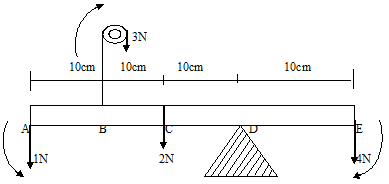# PHY3: MOMENTS

##### This Unit is about Moments as used in force and Physics in Olevel.

MOMENTS

A moment is concerned with the turning effort of a force. When two or more forces act at a point, they tend to make that body rotate for example opening a door, turning a tap, turning a nut using a spanner, turning the steering wheel of a car etc. the point about which the body turns is called the fulcrum or pivot. A very large turning effort can be achieved with comparatively small force provided the distance from the fulcrum is large. Magnitude of this turning effort is called a moment of force. Moment of force is the product of force and its perpendicular distance of its line of action from the turning point [pivot]. Moment of force = force x perpendicular distance from pivot. The S.1 unit of moment is the Newton meter [Nm]. Moment has both magnitude and direction hence is a vector. A moment of force may have a turning effect in dock wise [-] or on anti clockwise [+] direction

PRINCIPLES OF MOMENTS OF FORCE

When a body is in equilibrium, the sum of the anticlockwise – moments is about any point of the body equals to the sum of the clockwise moments about the point. E.g. A young boy of mass 25kg is playing with his sister of mass 40kg on a wooden plunk of length 5m revealed at exactly the centre if the boy sites’ 2m from the pivot, how for a way from the pivot should the sister be in order for the plunk to battle balance horizontally.Anti clockwise moment = F x d = 250 x 2 = 500N Clockwise moment = 400 x X = 400X , 400x=500. x = 5/4 X = 1.25m .If the forces below are in equilibrium, find force M20 x 40            +         10 x 30 800                     +       300 = 1100Ncm Clockwise moment = M x 10 = 400MNcmM = 110N

RESULTANT MOMENTS.

It is experienced when a body is acted open by many moments. Such a body may not be in equilibrium hence the existence of the resultant. It is conventional to give a positive sign to anti clockwise moment resultant. It is conventional to give a positive sign to a sign to anti clockwise moment and negative to a clockwise movement. The sign of a result moment will give its direction ExampleA rod AE of negligible weight 40cm long is pivoted at A and weight 1, 3, 2, 4, Newton’s act on the rod as shown in the diagram above. Find the resultant moment and its direction. Change units to S.1 and take moment about D Clockwise moment –                [4 x0.1] + – [3 x 0.2] – 0.4 – 0.6 = -1.0 Nm Anti clockwise [1 x 0.3] + [2 x 0.1] 0.30 + 0.20 = 0.5 Nm Result and moment = -1.0 + 0.5 = – 05Nm Resultant moments 0.5 Nm in the clockwise direction

THIS VIDEO IS ABOUT MOMENTS AND HOW THEY WORK

### ASSIGNMENT : MOMENTS assignmentMARKS : 10  DURATION : 1 week, 3 days

SEE ALL
•YOU